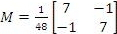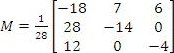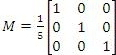Inverse Matrix

Inverse Matrix

Identity Matrix
Originally we have the identity property for a real number m, where

m1 = m and 1m = m

for any real number m. For a square matrix M, the identity property is found by using a square identity matrix I, such that

MI = M and IM = M

where I and M must be square matrices of the same size.

Example
Verify the identity property for matrices using matrix M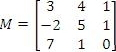MI = M

Therefore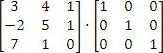Then we have each of the elements of the product:

(31) + (40) + (10) = 3
(30) + (41) + (10) = 4
(30) + (40) + (10) = 1

(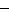1) + (50) + (10) =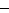(0) + (51) + (10) = 5
(0) + (50) + (11) = 1

(71) + (10) + (00) = 7
(70) + (11) + (00) = 1
(70) + (10) + (01) = 0

The resultant matrix is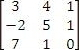The n x n identity matrix is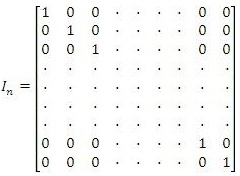Multiplicative Inverses
If M is an nn, matrix, its multiplicative inverse nn, has to satisfy the following properties:

MM -1 = In and M -1M = In

understanding two conditions, where

1.) Only a square matrix can have multiplicative inverse
2.) Even though m-1 = 1/m for any nonzero real number, M -1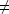1/M

Example
Find the inverse of matrix M: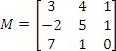we can say that the inverse of matrix M -1 would look like this: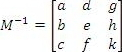Then using the inverse property we have

MM -1 = In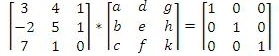3a + 4b + c = 1
3d + 4e + f = 0
3g + 4h + k = 0

-2a + 5b + c = 0
-2d + 5e + f = 1
-2g + 5h + k = 0

7a + b = 0
7d + e = 0
7g + h = 1

By solving for each of the variables we find the inverse matrix M -1: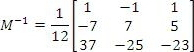Exercises

If possible find the inverse matrix for the following:
1)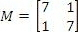2)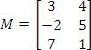3)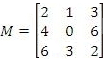4)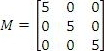--------------